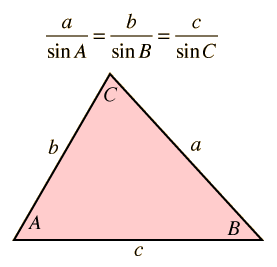# Law of Sines

The law of sines is used to find angles of a general triangle. If two sides and the enclosed angle are known, it can be used in conjunction with the law of cosines to find the third side and the other two angles.
 Click on the highlighted text for either angle A or angle C to initiate calculation.If two sides and the angle opposite one of them are specified, then the angle opposite the other can be calculated. The third angle is then determined by the fact that the sum of the interior angles of a triangle must equal to 180 degrees.
 Side a = Side b = Angle B = Angle A = Angle C = After doing the above calculation, the length of side c may be determined with the aid of the law of cosines. Side c =

### Applications

Index

 HyperPhysics*****HyperMath*****Trigonometry R Nave
Go Back

# Applications, Law of Sines

The law of sines has application to vector quantities:

It has application along with the law of cosines to the problem of the heading angle for an aircraft in the wind.

Index

 HyperPhysics*****HyperMath*****Geometry R Nave
Go Back